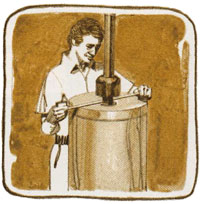Section 8: Program Editing126
instructions. If you specify a delete number that extends across the END of a program, the HP-41C only deletes up to the END of the program and stops. If you attempt to delete more lines than you have allocated, the calculator simply deletes lines up to the end of program memory or an END instruction and stops.
Let’s load a program into program memory and use the editing features to modify it.
To determine the heat loss from a cylindrical water heater, you need to know three things: the area of the cylinder, the convective heat transfer coefficient, and the temperature difference between the cylinder surface and the surrounding air. In the introduction to this handbook, you wrote a program (HEAT) that determined the heat loss from the water heater given the area, the heat transfer coefficient, and the temperature difference. In section 7, you wrote a program called CIRCLE to determine the surface area of one end of the cylinder.
 Now let’s write and load a program that determines the total surface area of the cylinder given its height (h) and radius (r). The formula used is S = (2 π r2) + (2 π r h). Below are the instructions for the program, assuming that the radius and the height have been placed into the X- and Y-registers of the stack, respectively. The name of the program is AREA.Keystrokes Display
PRGM
 00 REG 44
Places the HP-41C into program mode. HP-41C is positioned to the top of the previous program you executed.
g GTO · ·
 00 REG 44
Sets the HP-41C to the end of program memory and tells you the number or unused registers left in program memory.
g LBL
ALPHA AREA ALPHA
 01 LBLTAREA
Names the program.
STO 01
 02 STO 01
Stores the radius (r) into storage register R01.
g X2
 03 X 2